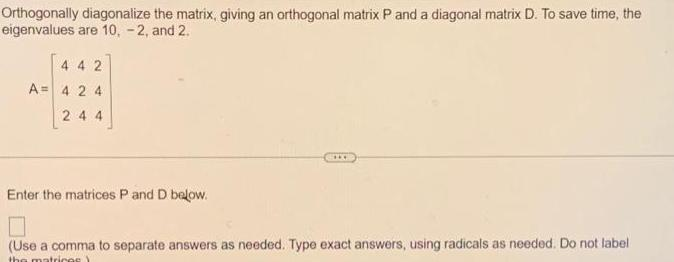Question:

# Orthogonally diagonalize the matrix giving an orthogonal

Last updated: 11/16/2023Orthogonally diagonalize the matrix giving an orthogonal matrix P and a diagonal matrix D To save time the eigenvalues are 10 2 and 2 442 A 424 244 Enter the matrices P and D below Use a comma to separate answers as needed Type exact answers using radicals as needed Do not label the matrices